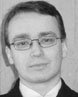‹ Instructors

# Roman OsipovRoman Osipov became a user of Mathematica in 2007 while working on his master of science diploma. He is now working on his PhD dissertation, which is devoted to numerical solving of nonlinear singular integral equations in Mathematica. He is an administrator of a Russian Mathematica user community and a Russian Mathematica VK group and he has created video lectures about Mathematica. He presented the report “Mathematical Design in Mathematica” at the Joint Institute for Nuclear Research (JINR) in Dubna, January 2011, and is the author of several scientific reports devoted to the application of Mathematica in applied mathematics, physics, chemistry, and education.

Osipov is a lecturer and an author of a Mathematica course for PhD students and scientists at Lomonosov MSU FCT and gives seminars and lectures for first- and second-year students on mathematical analysis, linear algebra, and ODE. He also gives seminars on operational calculus and complex analysis for undergraduate students. He enjoys helping to solve problems in Mathematica for a wide range of Russian-speaking users.

## Organization:

Lomonosov Moscow State University of Fine Chemical Technology (MSU FCT)

## Degrees:

MS in Applied Mathematics, Lomonosov MSU FCT

Russian, English

## Interests:

Family, programming, numerical methods, graphic design, mathematics in art, web development, helping other Mathematica users, complex analysis, differential and integral equations, A New Kind of Science, teaching, music of 1930s–60s, bodybuilding, bobsleigh, and much more